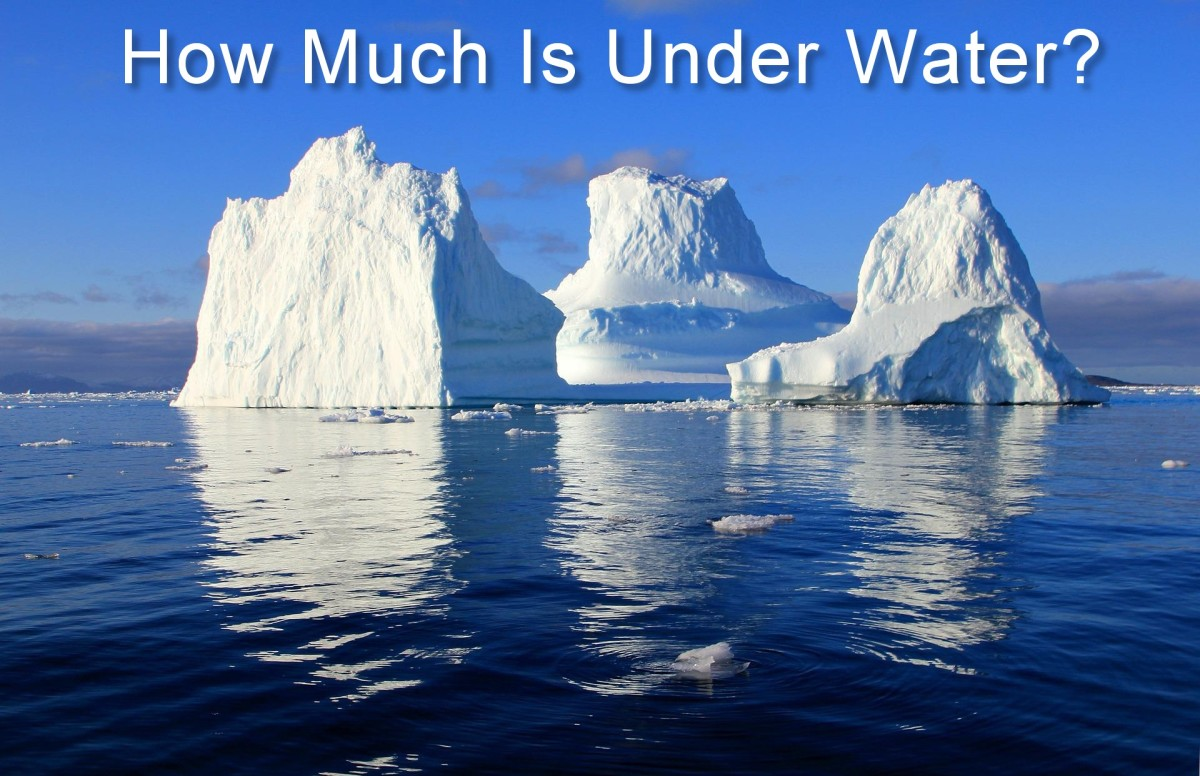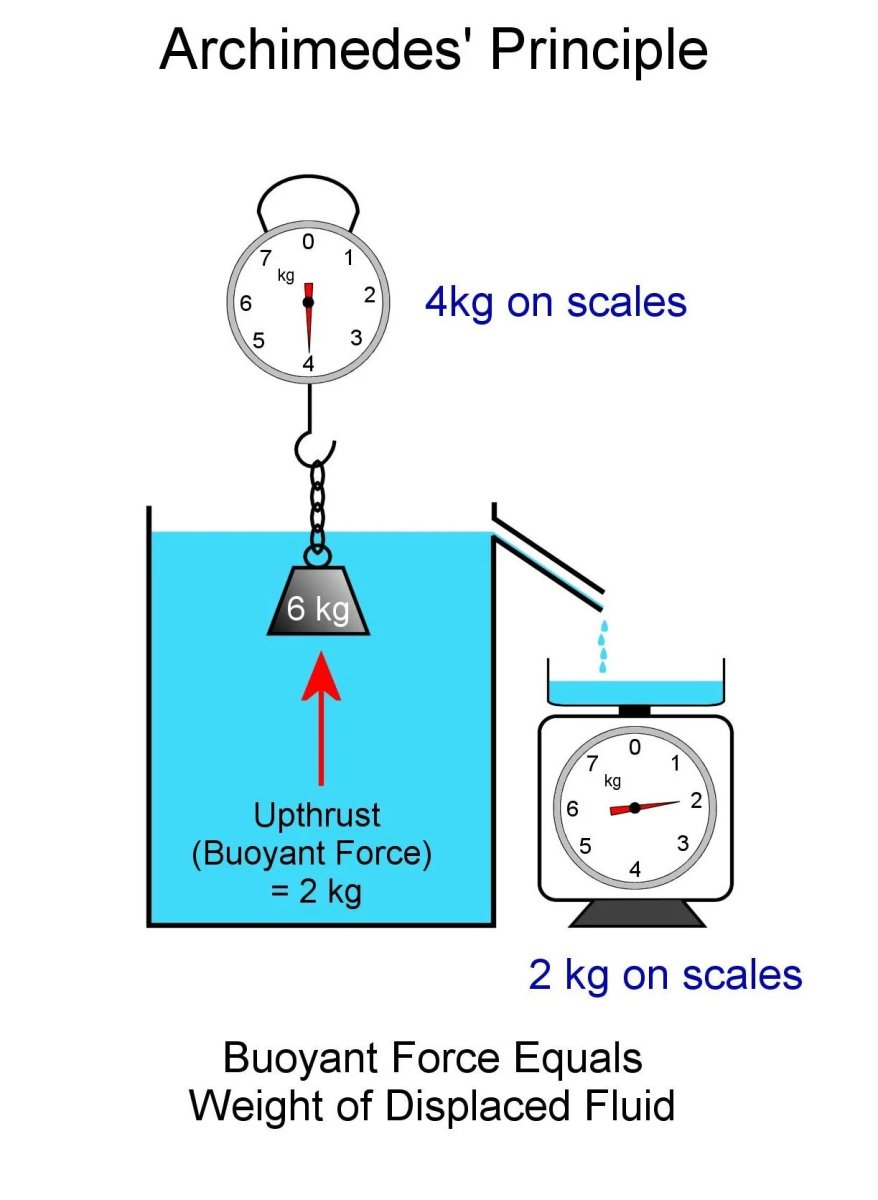# Why Is Most of an Iceberg Under Water? And How Much?

Eugene is a qualified control/instrumentation engineer Bsc (Eng) and has worked as a developer of electronics & software for SCADA systems.Read on to learn how much of an iceberg is underwater.Original uncaptioned image Lurens, public domain image via Pixabay.com

## Ninety Percent of an Iceberg Is Below the Waterline

Icebergs can be massive, but did you know that you're only seeing literally the tip of them above the water? Yes, up to 90% of an iceberg's volume can be under water. But why does that amount sink and not just float above sea level like the rest of it? In this article we explore why.

In a previous science tutorial called Archimedes' Principle, Buoyancy Experiments and Flotation Force, we discovered how objects placed in a liquid experience a force called buoyancy.

The Archimedean Principle or Principle of Archimedes simply states that when you put something into a fluid (A fluid can be a liquid like water or gas like air), the force acting upwards on the object equals the weight of fluid that was displaced. Imagine a container full to the brim with water. If something is put into the water in the container, it sinks a bit and floats, totally submerges and floats, or completely sinks to the bottom. The amount of water that flows over the brim is what is displaced.The principle of Archimedes. Buoyant force equals the weight of the displaced liquid. The weight measured by the scales is 6kg less 2 kg = 4 kg.© Eugene Brennan

## Working Out the Volume of an Iceberg That's Under the Water

In the image below, we have an iceberg with a lot of its volume under the water. To do the calculations, first let's assign some variables so that we can work out the equations.

V is the total volume of the iceberg

ρi is the density of fresh water ice

ρs is the density of seawater

k is the fraction of the berg that is underwater

g is the acceleration due to gravity

m is the mass of the displaced water

Wd is the weight of displaced water

Wi is the weight of the iceberg

Scroll to Continue

## Calculations

The total volume of the iceberg is V.

The variable k represents the proportion of the berg that is below the water. k has to be greater than or equal to 0 and less than or equal to 1. In other words, between none and all of the berg lies below the water line. We don't know what k is and it's this variable that we need to work out an equation for.

### Calculation of mass of displaced water

The volume of the berg below water = kV
and since the ice displaces its own volume, the volume of displaced water is also kV

The Archimedes Principle tells us that the upthrust or buoyancy force = weight of the displaced water. We need to work out the weight of this water.

We defined a variable ρs as the density of sea water

The volume of the displaced water = the volume of the berg below water = kV

We know that density = mass / volume, so mass = density x volume

So mass of the displaced water m = density of sea water x volume of water displaced.

m = ρskV .......................Eqn(1)

### Calculation of weight of displaced water

Now since Archimedes Principle specifies weight, we convert mass to weight by multiplying by the acceleration due to gravity

So weight = mg and tsubstituting for m from Eqn(1), the weight of displaced water

Wd = ρskVg .....................Eqn (2)

### Calculation of weight of iceberg

Ice sheets are made from snow that gets packed over long periods of time and converted into ice. Bits of ice eventually break off from the sheets and form floating icebergs. Since icebergs came from snow that originated as water that evaporated off land and sea, they're made of fresh water, not sea water. Pure fresh water has a density of 1 g/cm3. Ice is less dense than water however, because when water freezes, its volume increases. Since density = mass / volume and mass stays the same, but volume increases, density decreases. The density of ice ranges from 0.9167 to 0.9168 g/cm3

Assuming the berg is made of fresh water and its volume is V and the density of fresh water ice is ρi, then the weight Wi of the berg is

Wi = ρiVg .............................Eqn (3)

### Working out k, the proportion of the iceberg under water

Now put Eqn (2) and Eqn (3) together. Since the berg is floating, its weight acting downwards (A in the diagram above) is balanced by the upthrust (force B), equalling the weight of the displaced water)

So upthrust = Wd = weight of iceberg = Wi

Substituting for the values of Wd and Wi from Eqn (2) and Eqn (3) gives:

ρskVg = ρiVg

Simplifying by dividing both sides of the equation by Vg gives us

ρsk = ρi

or k = ρi / ρs

So now two things become apparent:

• The proportion k of the berg below water is independent of the size of the berg.
• k doesn't depend on the shape of the iceberg.

The proportion of an iceberg below sea level is simply the ratio of the density of fresh water ice to the density of sea water. If the density of ice is 0.9167 g/cm3 and the density of sea water is on average 1.025 g/cm3 . then

k = 0.9167 / 1.025 = 0.89.

So approximately nine tenths or 90% of an iceberg is under water.

## Why Is Most of an Iceberg Below Water?

We worked out the numbers mathematically above, but intuitively what is the reason? It's simply because the density of freshwater ice is so close to the density of seawater. So a lot of the iceberg has to sink below the surface to create enough displacement to generate the buoyancy force to balance the weight of the berg to keep it afloat.

If the density of ice equalled that of seawater, the berg would have to submerge completely to generate a buoyancy force to keep itself afloat. If the density was higher again (e.g., if it had lots of iron weights embedded inside), the weight of the displaced water would be less than the weight of the berg, even if fully submerged, and it would sink. If an object is made of styrofoam (expanded polystyrene), it only has to displace a little water in proportion to its volume to keep itself afloat.

## Sources and Further Reading

This content is accurate and true to the best of the author’s knowledge and is not meant to substitute for formal and individualized advice from a qualified professional.

© 2022 Eugene Brennan